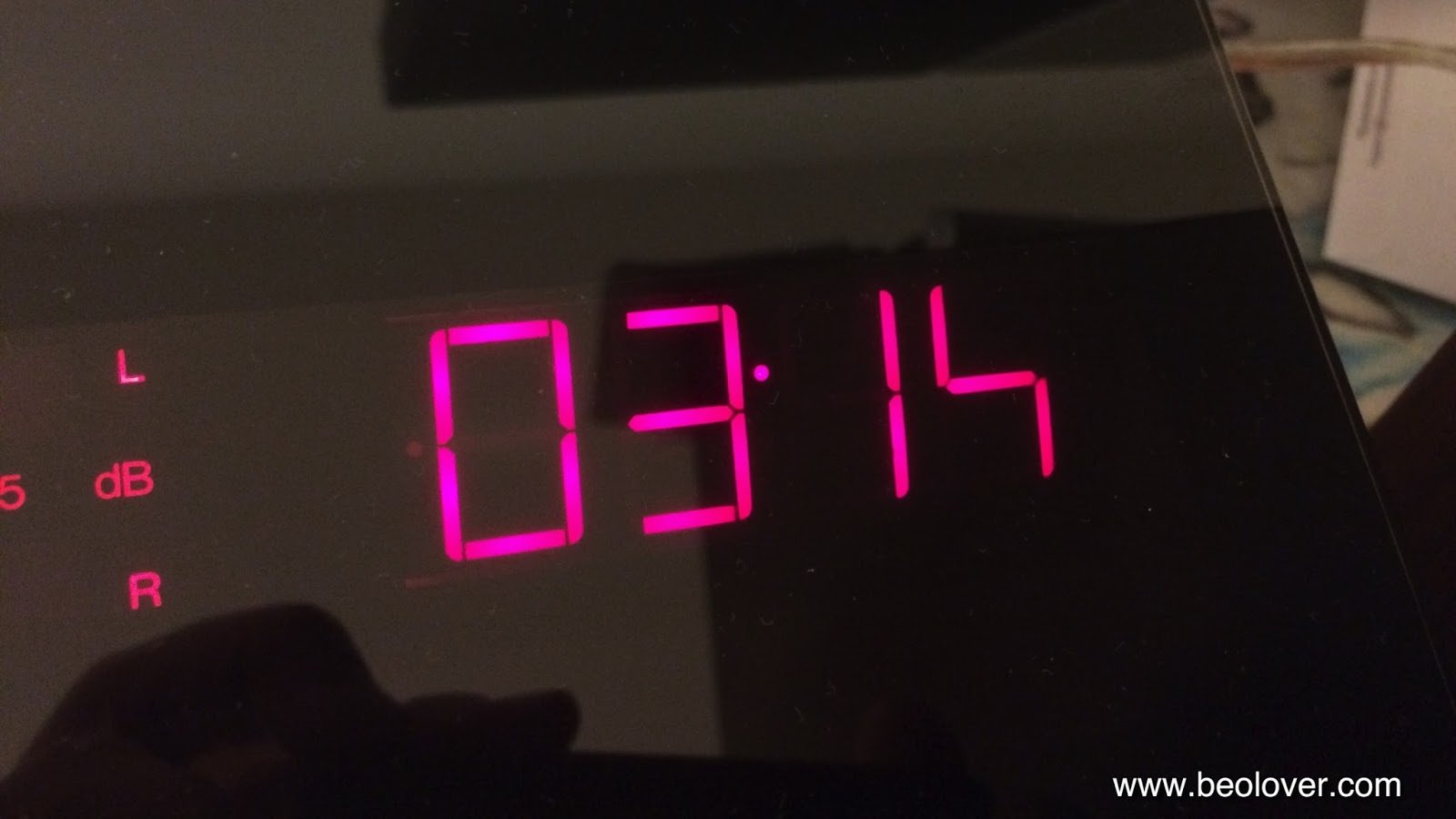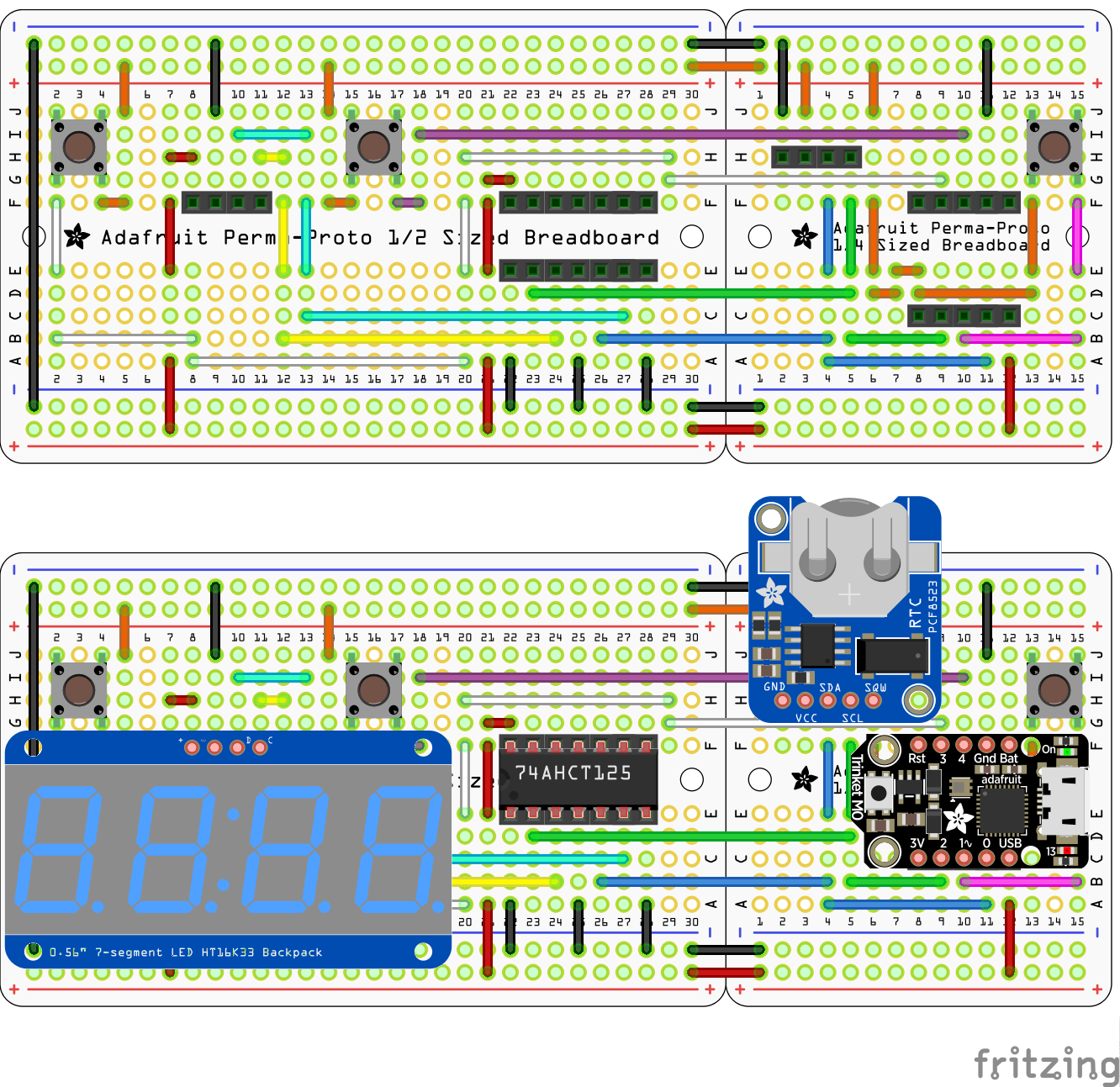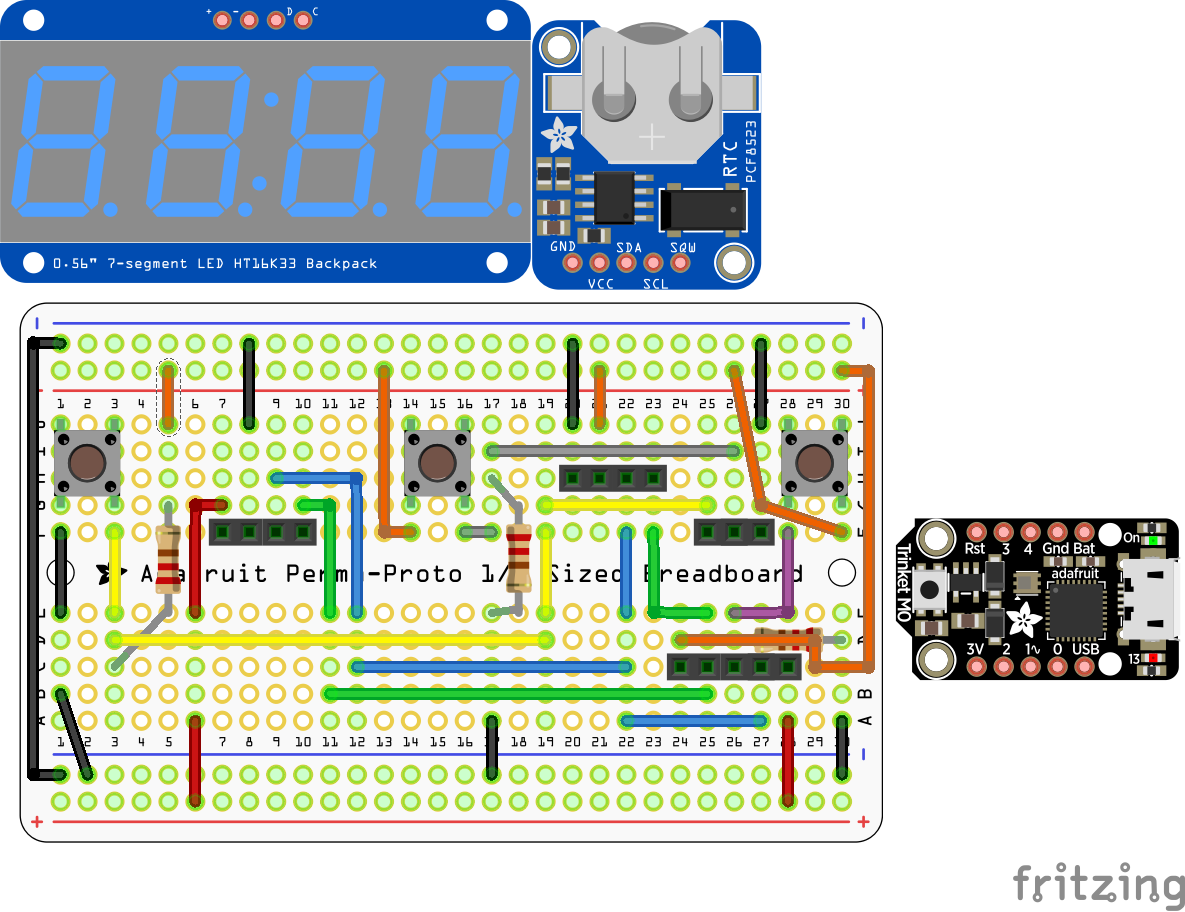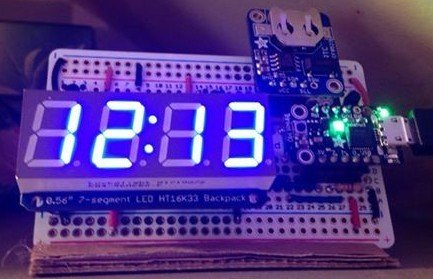### Leila

Last week we were at DjangoCon Europe in Heidelberg and I gave a lightning talk about a clock that we made with Python.

we made this clock because ours was broken, one of the segment was dead, like in this picture:After lot of research to find a new one with the right requirements (it had to be blue and it had to be visible when it was dark) and without finding what we were looking for we finally decided to make one ourselves.

## To make this clock we had to use:

• lots of wires
• an RTC module
• a blue quad 7-segment display and its driver
• an M0 trinket
• and few other stuff

Here is plan AThis didn't work because we used a 3.3V to 5V 1-way logic converter (the data sheet said the display drivers needed a 5V signal and the trinket outputs a 3.3V signal) but we should have used a 2-way logic converter (as the display driver "talks back" to the micro-controller) and we didn't have one.We had to make a plan B, after all the display driver works fine with a 3.3V signal, so we got rid of the logic converter all together and the whole circuit is that much simpler.

We also decided to use a single medium breadboard and therefore had to re-do the whole circuit.

## After the circuit the code

To make this clock we wrote 2 different files (written using mu)

• full_clock.py
• utils.py

### full_clock.py

full_clock.py is the main file of the project, it's used to read the time and date from the RTC module, display the time or what we want to display on the display and it's also used to manage buttons.

``````from board import SCL, SDA, D1, D3, D4
from busio import I2C

import digitalio

from time import sleep

from pcf8523_light import Rtc
from utils import bcd_to_int, int_to_bcd, get_dst

i2c = I2C(SCL, SDA)
rtc = Rtc(i2c)
display = Seg7x4(i2c)

# Initialize buttons
mode_btn = digitalio.DigitalInOut(D1)
mode_btn.direction = digitalio.Direction.INPUT
mode_btn.pull = digitalio.Pull.DOWN

left_btn = digitalio.DigitalInOut(D3)
left_btn.direction = digitalio.Direction.INPUT
left_btn.pull = digitalio.Pull.UP

right_btn = digitalio.DigitalInOut(D4)
right_btn.direction = digitalio.Direction.INPUT
right_btn.pull = digitalio.Pull.DOWN

debug = False

mode = 0
mode_ct = 0
max_ct = 100

)

write_mode_mapping = (
((Rtc.HOURS,  24, 0), (Rtc.MINUTES, 60, 0)),
((Rtc.MONTHS, 13, 1), (Rtc.DAYS,    32, 1)),
(None,                (Rtc.YEARS,  100, 0)),
)

old_r_val = 0
old_mode = 0

is_dst = False

while True:
if mode != 0:
mode_ct += 1

if cur is None:
continue

new_r_val = bcd_to_int(cur)
if old_r_val != new_r_val or old_mode != mode:
old_r_val = new_r_val
old_mode = mode

new_dst = get_dst(rtc)
if new_dst is not None:
is_dst = new_dst

# We only care about dst if displaying the time
if mode == 0 and is_dst:
cur = int_to_bcd((bcd_to_int(cur) + 1) % 24)

display.fill(0)

for i in range(2):
display.put('{}'.format(cur[i] & 0x0f), i * 2 + 1)
left = (cur[i] & 0xf0) >> 4
if left != 0 or (mode != 1 and i == 1):
# put expects a string
display.put('{}'.format(left), i * 2)

if mode == 0:
display.put(':')
elif mode == 1:
display.put('.', 1)

display.show()

if mode_btn.value:
mode = (mode + 1) % len(read_mode_mapping)
mode_ct = 0
sleep(.15)
else:
btn_index = 0 if left_btn.value == 0 else 1 if right_btn.value else -1
if btn_index != -1:
mode_ct = 0
write_operation = write_mode_mapping[mode][btn_index]

if write_operation is None:
continue

new_val = int_to_bcd((bcd_to_int(cur[btn_index]) + 1) % write_operation)

if new_val == 0:
new_val += write_operation

if debug:
print(cur[btn_index], bcd_to_int(cur[btn_index]), '->', new_val)

rtc.write(write_operation, new_val, debug)
old_r_val = 0xff
sleep(.15)

if mode_ct > max_ct:
mode = 0

sleep(.1)
``````

### utils.py

utils.py is used to pass from binary to integer and back it's also used to determine if it's summer or winter time.

`get_dst` is the daylight-saving-time rule for Western Europe because we are limited in memory and the clock is located in Belgium and we have no intention of moving it in the near future.

``````def int_to_bcd(val):
return ((val // 10) << 4) + (val % 10)

def bcd_to_int(val):
return ((val & 0xf0) >> 4) * 10 + (val & 0x0f)

def get_dow(day, month, year, debug=False):
Y = year if month > 2 else year - 1
y = Y % 100
c = Y // 100
m = (month - 2) if month > 2 else month + 10

if debug:
print(Y, y, c, m)

rv = int(day + (2.6 * m - .2) + y + (1.0 * y) / 4 + (1.0 * c) / 4 - 2 * c) % 7

if debug:
print('DOW for {}-{}-{} is {}'.format(year, month, day, rv))

return rv

def get_dst(rtc, debug=False):

if not all(today):
return None

today = [bcd_to_int(item) for item in today]
if today > 10 or today < 3:
if debug:
print('Winter')
return False
if today > 3 and today < 10:
if debug:
print('Not winter')
return True
if today < 25:
print('Early in month')

if year is None:
return None

year = 2000 + bcd_to_int(year)
dow = get_dow(today, today, year)

if dow == 0:
if debug:
print('Sunday')
return (today == 3 and hour >= 2) or (today == 10 and hour < 2)

dow_25 = get_dow(25, today, year)
if dow_25 == 0:
dow_25 = 7

if debug:
print(dow, dow_25)

return (dow < dow_25) ^ (today == 10)
``````

## Final clock

In the end, after a week of work we finished the clock, here is what it looks like: